# 一类时滞交通流模型的多稳定性研究Multistability in Delay Car-Following Models

DOI: 10.12677/DSC.2019.84030, PDF, HTML, XML, 下载: 284  浏览: 410  国家自然科学基金支持

Abstract: It is well-known that traffic system is a complex nonlinear large system. There are a great number of factors to influence the evolutionary behavior of the system. It is a challenging job to propose a mathematical model to accurately describe such a real system. In car following models of traffic dynamics, there exists a finite time for human drivers to process stimuli of preceding vehicles and make a decision. It is natural to give a detailed study of the mechanism about how time delays affect the traffic flow patterns and their evolutions. In this paper, we are interested in rich dynamical behavior in a class of nonlinear car-following models, including stability, Hopf bifurcations, multistability and chaotic behavior etc. Numerical simulation gives a solid verification of our findings.

1. 引言

${\stackrel{¨}{x}}_{i}\left(t+\tau \right)=\frac{a{\stackrel{˙}{x}}_{i}^{m}\left(t\right)\left[{\stackrel{˙}{x}}_{i-1}\left(t\right)-{\stackrel{˙}{x}}_{i}\left(t\right)\right]}{{\left[{x}_{i-1}\left(t\right)-{x}_{i}\left(t\right)\right]}^{l}}+b\left[{x}_{i-1}\left(t\right)-{x}_{i}\left(t\right)-{L}_{i}\right]$ (1)

2. 模型描述与多稳定性分析

$\begin{array}{l}\text{d}y/\text{d}t=\left[{v}_{1}\left(t\right);-a{v}_{1}\left(t-\tau \right)/{d}_{1}\left(t-\tau \right)-b\left({d}_{1}\left(t-\tau \right)-{L}_{1}\right);{v}_{2}\left(t\right);a{v}_{\text{1}}\left(t-\tau \right)/{d}_{\text{1}}\left(t-\tau \right)\\ \text{\hspace{0.17em}}\text{\hspace{0.17em}}\text{\hspace{0.17em}}\text{\hspace{0.17em}}\text{\hspace{0.17em}}\text{\hspace{0.17em}}\text{\hspace{0.17em}}\text{\hspace{0.17em}}\text{\hspace{0.17em}}\text{\hspace{0.17em}}\text{ }\text{\hspace{0.17em}}+b\left({d}_{\text{1}}\left(t-\tau \right)-{L}_{\text{1}}\right)-a{v}_{\text{2}}\left(t-\tau \right)/{d}_{\text{2}}\left(t-\tau \right)-b\left({d}_{\text{2}}\left(t-\tau \right)-{L}_{\text{2}}\right)\right]\end{array}$ (2)

$\begin{array}{l}\text{d}y/\text{d}t=\left[{v}_{1}\left(t\right);-a{v}_{1}\left(t-\tau \right)/{d}_{1}\left(t-\tau \right)-b\left({d}_{1}\left(t-\tau \right)-{L}_{1}\right)-k{\left({d}_{\text{1}}\left(t\right)-{L}_{\text{1}}\right)}^{\text{5}};{v}_{2}\left(t\right);\\ \text{\hspace{0.17em}}\text{\hspace{0.17em}}\text{\hspace{0.17em}}\text{\hspace{0.17em}}\text{\hspace{0.17em}}\text{\hspace{0.17em}}\text{\hspace{0.17em}}\text{\hspace{0.17em}}\text{\hspace{0.17em}}\text{\hspace{0.17em}}\text{\hspace{0.17em}}\text{\hspace{0.17em}}a{v}_{\text{1}}\left(t-\tau \right)/{d}_{\text{1}}\left(t-\tau \right)+b\left({d}_{\text{1}}\left(t-\tau \right)-{L}_{\text{1}}\right)-a{v}_{\text{2}}\left(t-\tau \right)/{d}_{\text{2}}\left(t-\tau \right)\\ \text{\hspace{0.17em}}\text{\hspace{0.17em}}\text{\hspace{0.17em}}\text{\hspace{0.17em}}\text{\hspace{0.17em}}\text{\hspace{0.17em}}\text{\hspace{0.17em}}\text{\hspace{0.17em}}\text{\hspace{0.17em}}\text{\hspace{0.17em}}\text{\hspace{0.17em}}\text{\hspace{0.17em}}-b\left({d}_{\text{2}}\left(t-\tau \right)-{L}_{\text{2}}\right)+k{\left({d}_{\text{1}}\left(t\right)-{L}_{\text{1}}\right)}^{\text{5}}-k{\left({d}_{\text{2}}\left(t\right)-{L}_{\text{2}}\right)}^{\text{5}}\right]\end{array}$ (3)

${\tau }_{j}^{*}=\frac{\mathrm{arctan}\frac{a\omega }{b{L}_{i}}+\pi j}{\omega },\text{\hspace{0.17em}}j=0,1,2,\cdots$

$\omega =\sqrt{\frac{{a}^{2}+\sqrt{{a}^{4}+4{L}_{i}^{4}{b}^{2}}}{2{L}_{i}^{2}}},\text{\hspace{0.17em}}\text{\hspace{0.17em}}i=1,2.$

${\Re \frac{\text{d}\lambda }{\text{d}\tau }|}_{\tau ={\tau }_{j}^{*}}=\frac{-a{\omega }^{2}\left(a-b{L}_{i}\tau -2{L}_{i}\omega \mathrm{sin}\left(\omega \tau \right)\right)+{L}_{i}b{\omega }^{2}\left(2{L}_{i}\mathrm{cos}\left(\omega \tau \right)-a\tau \right)}{{\left(a-b{L}_{i}\tau -2{L}_{i}\omega \mathrm{sin}\left(\omega \tau \right)\right)}^{2}+{\omega }^{2}{\left(2{L}_{i}\mathrm{cos}\left(\omega \tau \right)-a\tau \right)}^{2}},$

${\Re \frac{\text{d}\lambda }{\text{d}a}|}_{\tau ={\tau }_{j}^{*}}=\frac{-{\omega }^{2}\left(2{L}_{i}\mathrm{cos}\left(\omega \tau \right)-a\tau \right)}{{\left(a-b{L}_{i}\tau -2{L}_{i}\omega \mathrm{sin}\left(\omega \tau \right)\right)}^{2}+{\omega }^{2}{\left(2{L}_{i}\mathrm{cos}\left(\omega \tau \right)-a\tau \right)}^{2}},$

${\Re \frac{\text{d}\lambda }{\text{d}b}|}_{\tau ={\tau }_{j}^{*}}=\frac{-\left(a-b{L}_{i}\tau -2{L}_{i}\omega \mathrm{sin}\left(\omega \tau \right)\right)}{{\left(a-b{L}_{i}\tau -2{L}_{i}\omega \mathrm{sin}\left(\omega \tau \right)\right)}^{2}+{\omega }^{2}{\left(2{L}_{i}\mathrm{cos}\left(\omega \tau \right)-a\tau \right)}^{2}}.$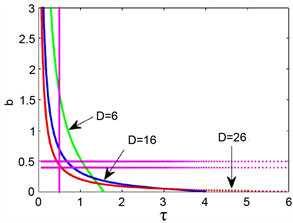(a)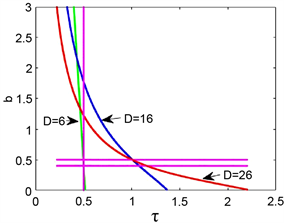(b)

Figure 1. Critical values of losing stability by (double) Hopf bifurcations in system (2) as b varies and a is fixed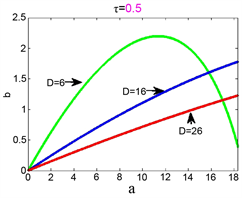(a)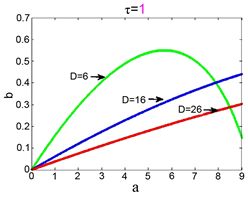(b)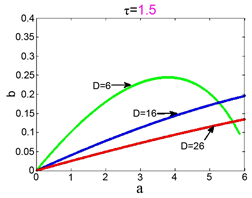(c)

Figure 2. Critical values of losing stability by (double) Hopf bifurcations in system (2) as τ varies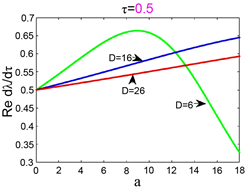Figure 3. Transversality conditions

3. 进一步分析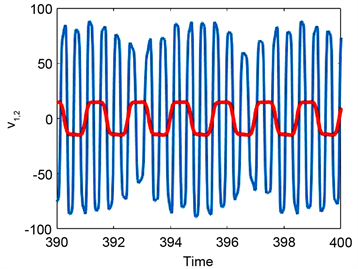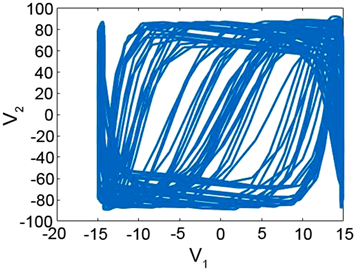(a)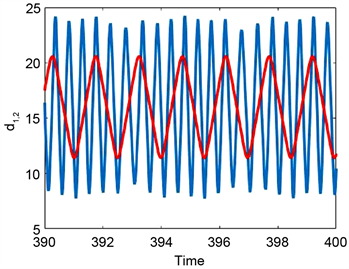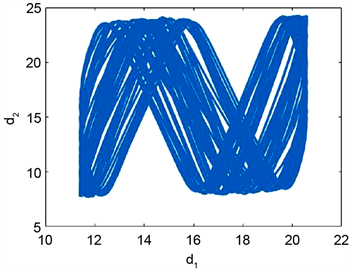(b)

Figure 4. Chaotic behavior in system (3) with a = 6, b = 0.8, L1 = 16, L2 = 16, τ = 0.99,k = 0.08 under the initial condition $y\left(t\right)\equiv {\left[\begin{array}{cccc}16.7& 0.01& 17.9& 0.01\end{array}\right]}^{\text{T}}$ , $t\in \left[-\tau ,0\right]$ . (a) Time series; (b) Phase portrait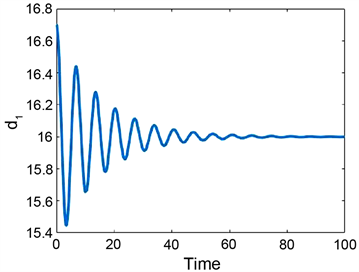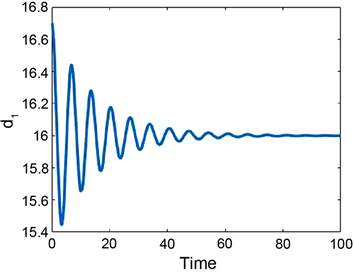(a)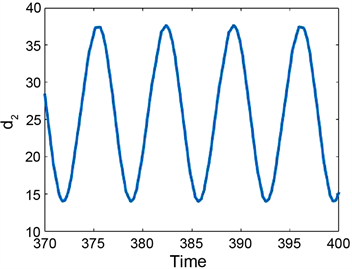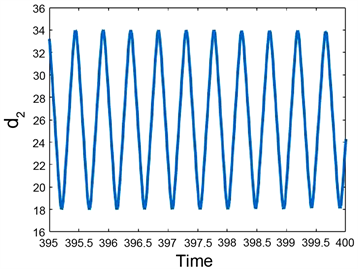(b)

Figure 5. Multistability in system (2) and (3) for a = 6, b = 0.8, L1 = 16, L2 = 26, τ = 0.3 under the initial condition $y\left(t\right)\equiv {\left[\begin{array}{cccc}16.7& 0.01& 17.9& 0.01\end{array}\right]}^{\text{T}}$ , $t\in \left[-\tau ,0\right]$ . (a) System (3.2), k = 0; (b) System (3.3), k = 0.08

4. 结论

  徐鉴, 徐荣改. 时滞车辆跟驰模型及其分岔现象[J]. 力学进展, 2013(1): 29-38.  Sun, J., Zheng, Z. and Sun, J. (2018) Stability Analysis Methods and Their Applicability to Car-Following Models in Conventional and Connected Environments. Transportation Research Part B, 109, 212-237. https://doi.org/10.1016/j.trb.2018.01.013  凌代俭, 肖鹏. 一类非线性车辆跟驰模型的稳定性与分岔特性[J]. 交通运输学报, 2009, 7(4): 6-11.  Nickerson, K.W. (1973) Biological Functions of Multistable Proteins. Journal of Theoretical Biology, 40, 507-515. https://doi.org/10.1016/0022-5193(73)90007-6  Safonov, L.A., Tomer, E., et al. (2002) Multifractal Chaotic Attractors in a System of Delay-Differential Equations Modeling Roadtraffic. Chaos, 12, 1006-1014. https://doi.org/10.1063/1.1507903  Das, S.L. and Chatterjee, A. (2002) Multiple Scales without Centermanifold Reductions for Delay Differential Equations nearHopf Bifurcations. Nonlinear Dynamics, 30, 323-335. https://doi.org/10.1023/A:1021220117746  Faria, T. (1995) Normal Forms for Retarded Functional Differential Equations with Parameters and Applications to Hopfbifurcation. Journal of Differential Equations, 122, 181-200. https://doi.org/10.1006/jdeq.1995.1144  Guo, S. and Man, J. (2013) Patterns in Hierarchical Networks of Neuronal Oscillators with Symmetry. Journal of Differential Equations, 254, 3501-3529. https://doi.org/10.1016/j.jde.2013.01.027  Xu, J., Chung, K.-W. and Chan, C.-L. (2007) An Efficient Method for Studying Weak Resonant Double Hopf Bifurcation in Nonlinear Systems with Delayed Feedback. SIAM Journal on Applied Dynamical Systems, 6, 29-60. https://doi.org/10.1137/040614207